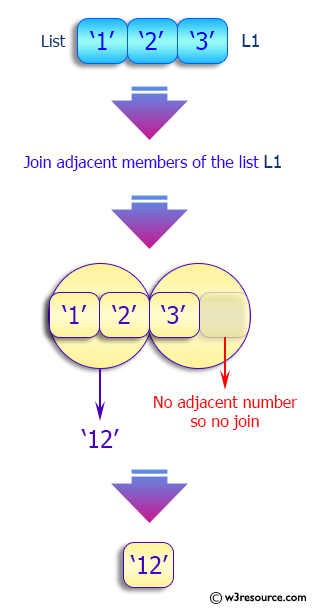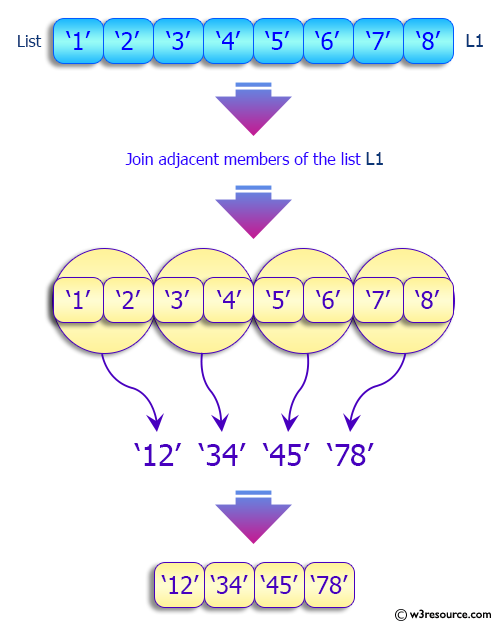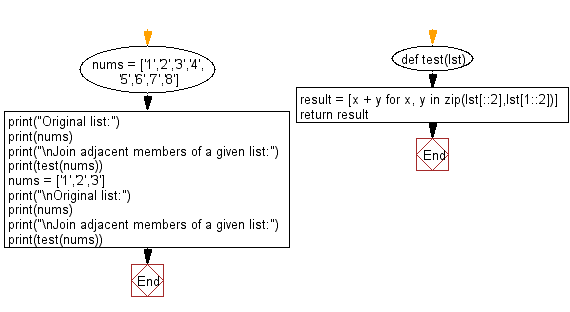﻿ Python: Join adjacent members of a given list - w3resource# Python: Join adjacent members of a given list

## Python List: Exercise - 203 with Solution

Write a Python program to join adjacent members of a given list.

Sample Solution:

Python Code:

``````def test(lst):
result = [x + y for x, y in zip(lst[::2],lst[1::2])]
return result

nums = ['1','2','3','4','5','6','7','8']
print("Original list:")
print(nums)
print("\nJoin adjacent members of a given list:")
print(test(nums))

nums = ['1','2','3']
print("\nOriginal list:")
print(nums)
print("\nJoin adjacent members of a given list:")
print(test(nums))
```
```

Sample Output:

```Original list:
['1', '2', '3', '4', '5', '6', '7', '8']

Join adjacent members of a given list:
['12', '34', '56', '78']

Original list:
['1', '2', '3']

Join adjacent members of a given list:
['12']
```

Pictorial Presentation:Flowchart:## Visualize Python code execution:

The following tool visualize what the computer is doing step-by-step as it executes the said program:

Python Code Editor:

Have another way to solve this solution? Contribute your code (and comments) through Disqus.

What is the difficulty level of this exercise?

Test your Python skills with w3resource's quiz

﻿

## Python: Tips of the Day

```print(2_000_000)
```2000000